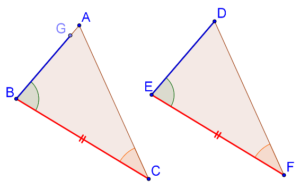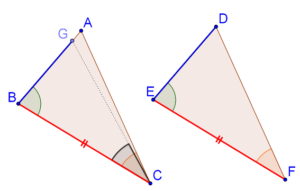# The ASA Criterion Proof

The ASA Criterion Proof

## What is ASA congruence criterion?

ASA congruence criterion states that if two angle of one triangle, and the side contained between these two angles, are respectively equal to two angles of another triangle and the side contained between them, then the two triangles will be congruent.

Note: Refer ASA congruence criterion to understand it in a better way.

## ASA congruence criterion Proof:

For this purpose, consider $$\Delta ABC$$ and $$\Delta DEF$$, where $$BC = EF$$, $$\angle B = \angle E$$ and $$\angle C = \angle F$$. Note that this will also mean that $$\angle A = \angle D$$ (can you see why?). Now, we have to show that these two triangles are congruent. Here is a step-by-step proof.

Step 1: If $$AB$$ is equal to $$DE$$, then the two triangles would be congruent by the SAS congruence criterion. So, let us suppose that $$AB$$ is not equal to $$DE$$. Then, one of them would be greater than the other – say that $$AB$$ > $$DE$$.

Note: Refer SAS congruence criterion to understand Step 1.

Step 2: Mark a point on $$AB$$ (call it $$G$$), such that $$GB = DE$$, as shown below:Step 3: We note that $$\Delta GBC$$ is congruent to $$\Delta DEF$$ by the SAS criterion. This means that $$\angle BCG = \angle F$$.Step 4: Finally, we note that $$\angle F = \angle C$$ (given), and so $$\angle BCG = \angle C$$.Think: Is that possible if $$CG$$ is in a different direction than $$CA$$?

No, it’s not!

This means that $$CG$$ must be in the same direction as $$CA$$, or in other words, $$G$$ and $$A$$ coincide, or: $$GB = AB = DE$$. Thus, the two triangles are congruent by the SAS congruence criterion.

Note: An important aspect of the proof of the ASA congruence criterion we have encountered is that it builds on the SAS congruence criterion – it assumes the truth of the SAS congruence criterion.